Next: 6.2.6 Recall Up: 6.2 Methods Previous: 6.2.4 Tuning curves

## 6.2.5 Training

In training, the local PCA mixture models NGPCA, NGPCA-constV, and
MPPCA-ext were used (chapter 3). On the pattern association task, they were compared to kernel PCA (chapter 5), to a look-up table, and to a multi-layer perceptron. All of them used the same preprocessed pattern set.

Section 3.3 mentioned that MPPCA-ext comprises the following two modifications: a correction for `empty' units and the use of an on-line PCA algorithm, which allows that noise can be added to each presented training pattern. On the visuomotor data, these two modifications turned out to be essential because otherwise, the algorithm became numerically unstable; some eigenvalues dropped to zero.

For the mixture models, 120 units and four principal components were used. The number of principal components was chosen after inspecting the local dimensionality of the pattern distribution. As described in section 4.5.1, the ratio of successive eigenvalues, averaged from a PCA in the neighborhood of each training pattern, has a peak at the local dimensionality of the distribution (Philipona et al., 2003). On the collected data, the first peak is at three (figure 6.7, left). This matches the expectation, since the brick had three degrees of freedom: two for the position and one for the orientation.

Figure 6.7: (Left) Ratio of successive averaged eigenvalues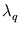and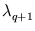. (Right) Illustration that additional principal components (here, one in y-direction) can account for the additional variance that results from the manifold's curvature.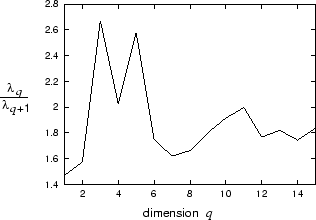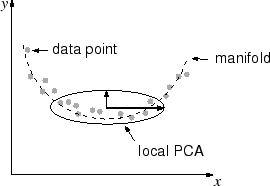Different from the kinematic arm model in section 4.5, however, figure 6.7 shows a second peak at five dimensions. The reason is probably that in the real robot task, the neighborhood of a pattern also covers the turns and twists of the underlying manifold because the data are much more sparse (3371 training patterns lie in a 68-dimensional space, compared to 50 000 patterns in ten dimensions for the kinematic arm model). A turn increases the local variance (figure 6.7, right). Therefore, for the mixture model, four principal components were chosen instead of three (this also improved the performance).

For NGPCA and NGPCA-constV, two sets of training parameters were used. Set 1 had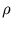(0) = 1.0,(tmax) = 0.00001,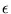(0) = 0.1,(tmax) = 0.01, and tmax = 400 000. Set 2 had the same parameters as the kinematic arm model (section 4.5):(0) = 10.0,(tmax) = 0.0001,(0) = 0.5,(tmax) = 0.001, and tmax = 400 000.

Kernel PCA extracted 150 eigenvectors and used an inverse-multiquadratic kernel with width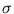= 7.0 (see section 2.4.3). In the 68-dimensional space, distances are larger than in the previous applications (chapter 5). Thus, the inverse-multiquadratic function was of advantage since it does not decline as quickly as a Gaussian function. Moreover, a reduced set with m = 1000 was used (appendix B.2).

The look-up-table method chooses a pattern from the training set whose visual part has the smallest Euclidean distance to the presented input. The multi-layer perceptron maps the visual part to the postural part. A structure with one hidden-layer, containing 20 neurons, was used. The hidden neurons had sigmoid activation functions. The weights were initialized with random values drawn uniformly from the interval [- 0.5;0.5]. For training, 3 000 epochs of resilient propagation were used (Riedmiller and Braun, 1993).Next: 6.2.6 Recall Up: 6.2 Methods Previous: 6.2.4 Tuning curves
Heiko Hoffmann
2005-03-22